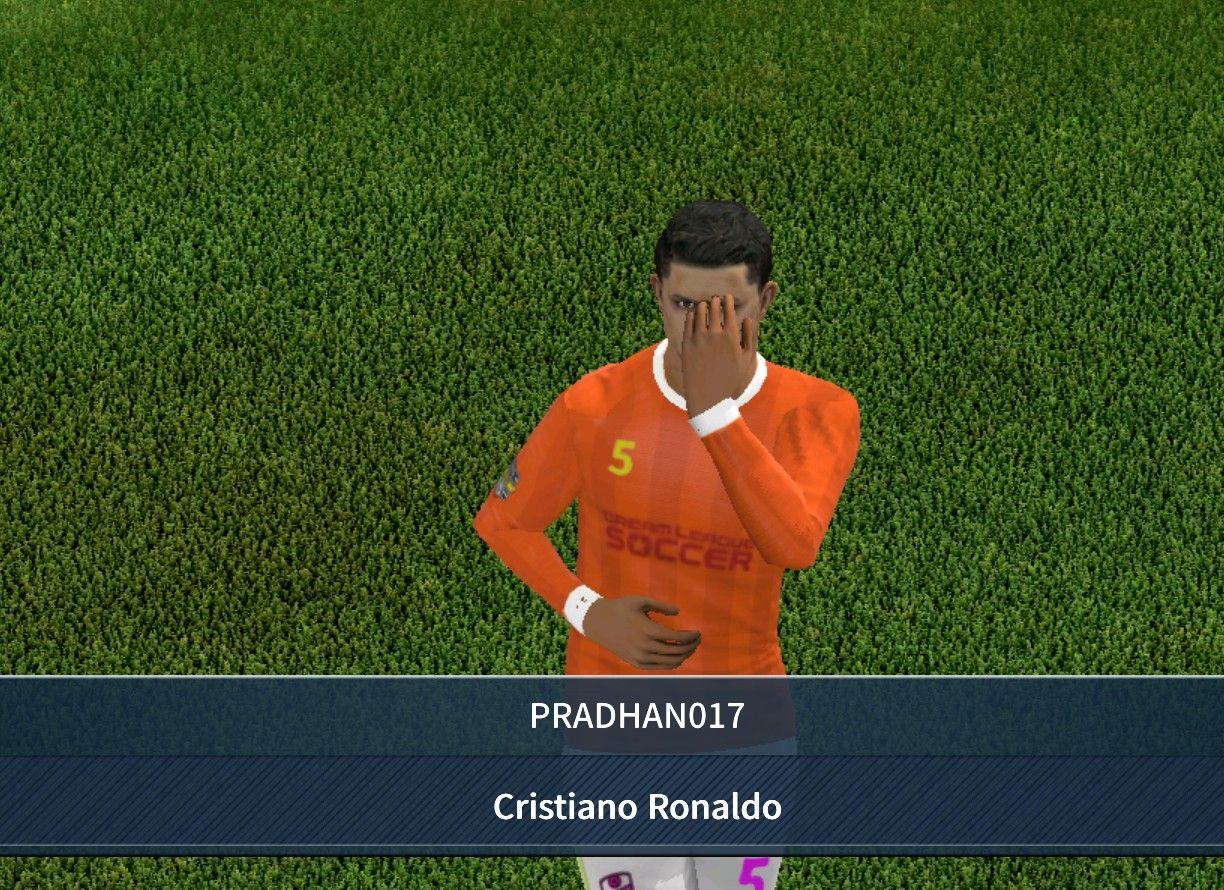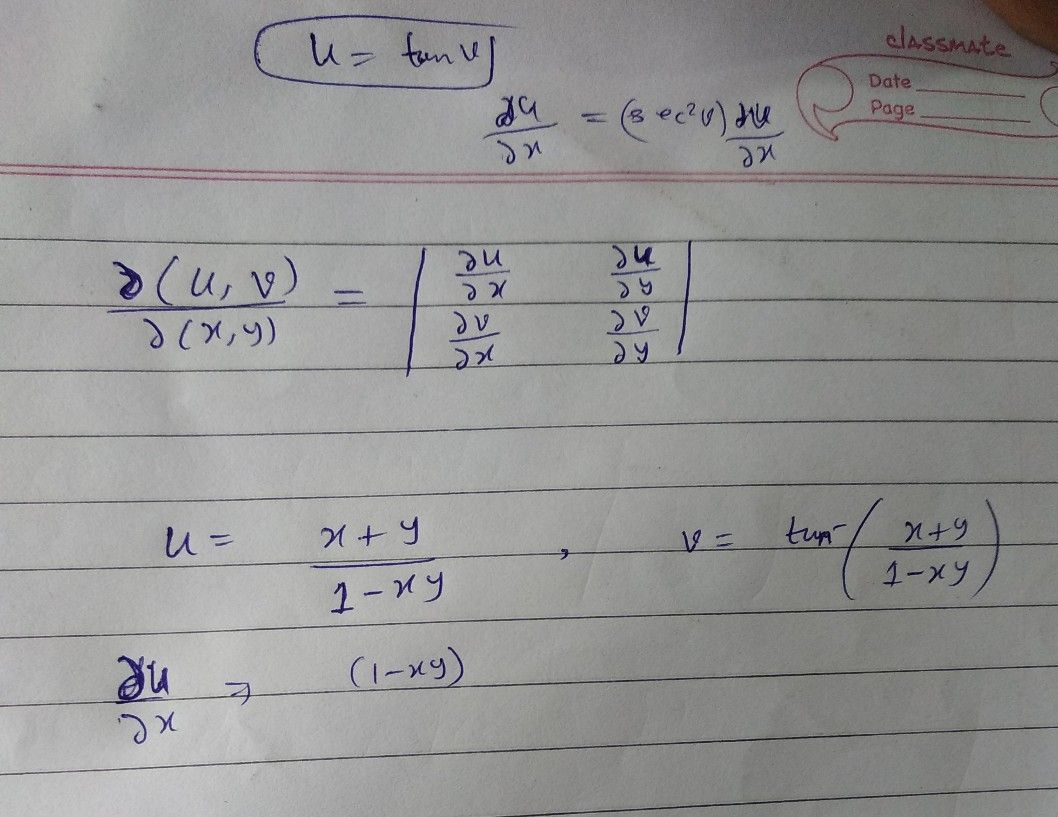Symbol
Problem$\left(1.\right)2$ *... $\left(11\right)$ Prove that $0\left(x_{1,x_{2}}$ $\dfrac {n^{\right)}} {x_{n}\right)}$ $\dfrac {y\left(x_{1.x_{2}}} {δ\left(y_{1.y2}}$ $\dfrac {x_{n}\right)} {y_{n}\right)}$ = 1. 22 If $y=x^{2}+y^{2}+x^{2},y=x+y+2.m=xy+yz+2x$ show that the Jacobian $\dfrac {d\left(n,v,y\right)} {0\left(x,y.z\right)}$ vanishes identically. Also finG between van $36$ $1$ $a=\left(x+y\right)/\left(1-x\right)\right)$ $4nd$ $y=1an^{-1}x+tan$ y, find $\dfrac {\left(y,y\right)} {0\left(x,y\right)}$ $△re$ u and v functionallyrelated $24sQ$ so find the relationship. If the functions $14y$ w of three independent variables x, y, z are not prove that the Jacobian of u, v, w with respect to x, y, z vanishes. 25 Show that the functions $=3x+2y-2,y=x-2y+2$ $4nd$ w x not independent and find the relation between them. 26. Show that the functions
Calculus
SolutionQanda teacher - BKMSir